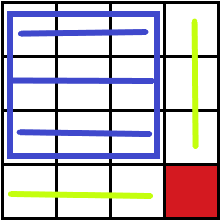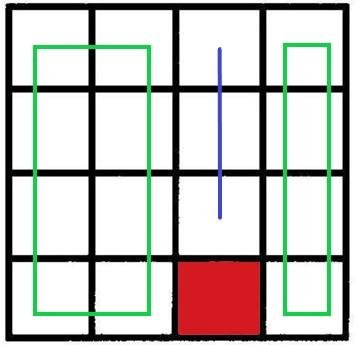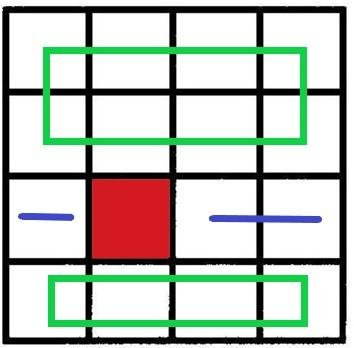# checkerboard problem

Home Forums Math Olympiad, I.S.I., C.M.I. Entrance checkerboard problem

Tagged: , , ,

Viewing 3 posts - 1 through 3 (of 3 total)
• Author
Posts
• #57158

You are given a 4 × 4 chessboard, and asked to fill it with five 3 × 1 pieces and one 1 × 1 piece. Then, over all such fillings, the number of squares that can be occupied by the 1 × 1 piece is
(A) 4 (B) 8 (C) 12 (D) 16

#57343

will get back to you in 24 hours

#57541

3 cases may arise

Case 1 :

Placing the $1\times 1$ piece at any corner of the board.

then there we can place  $3\times 1$ piece in that row or column .

then 7 square are filled and a $3\times 3$ square left to fill which can be easily filled by 3 $3\times 1$ pieces.Case 2 :

Placing $1 \times 1$ piece at any square on the edge except the corner squares.

then it is dividing that edge in $2 boxes : 1 box$ ratio. then this edge can not be filled with $3\times 1$ pieces.

Let us divide the board in two rectangular parts of dimensions $4\times 2$  and $4\times 1$ by the row (or column) of  $1\times 1$ piece . Those two rectangular parts can not be covered with $3\times 1$ pieces.Case 3 :

$1\times 1$ square is places in any middle square.

then the row (or column) is divided in the ratio $2 squares : 1 squares$ which can not be covered with $3\times 1$ pieces.

Let us divided the board into two rectangular parts dimensions $4\times 2$  and $4\times 1$ by the row (or column) of $1\times 1$ piece.

Those rectangles can not be covered with $3\times 1$ pieces.Viewing 3 posts - 1 through 3 (of 3 total)
• You must be logged in to reply to this topic.